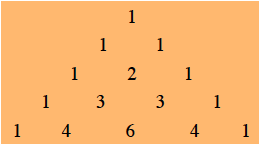### Home > PC > Chapter 7 > Lesson 7.3.3 > Problem7-121

7-121.

Use Pascal’s triangle to expand $(a + bc)^4$. Hint: Expand $(x + y)^4$ and then substitute the $a$ for $x$ and $bc$ for $y$.

Use Pascal's triangle to get the coefficients quickly.Substitute $(bc)$ for $y$ with the ( ).
You will make fewer careless errors!